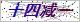网站首页  ◇  产品展示    ◇   ◇   仪表备品备件 > Z695=004=004校准泵/冲洗泵
•••••# 校准泵/冲洗泵

Products

### 产品概述

Z-695=004=004型   校准泵/冲洗泵695=004=004校准泵/冲洗泵是国外KNF一家公司制造商生产的产品，配套与9210硅表/9211磷表/9245钠表使用。9210硅酸根/磷酸根分析仪备件

 序号 名称 订货号 1 接口板 09200=A=5000 2 9210硅表CPU板带显示 09200=A=1000 3 9211磷表CPU板带显示 09200=A=1011 4 电源板 09200=A=2000 5 RS485板（MODBUS） 09125=A=0485/2485 6 92XX内部总线通讯板 09200=A=5500 7 2米内部连接电缆/4米/7米 370=302=034/304/307 8 921X测量板（0~1000ppb-SiO2/0~5ppm-PO4） 0921X=A=1500 9 9210硅表测量板（0~5000ppb-SiO2） 09210=A=1510 10 9211磷表测量板（0~50ppm-PO4） 09210=A=1520 11 管子4×6（国内制定） 12 样水电磁阀,NPS0,8（0,2~6bar） 689=118=008 13 O-型圈（流速调节螺钉）,4×1mm 35600905040 14 样水流速调节螺钉 09210=A=0105 15 样水液位检测器 09210=A=0250 16 O-型圈（液位检测器）,38×2mm 35609935308 17 样水电磁阀,（溢流容器） 689=118=024 18 921X连接板（混合器/加热器） 09210=A=1234 19 921X混合器 09210=A=0400 20 磁性搅拌棒 226=003=013 21 加热元件 09097=C=0560 22 Pt100温度探头 35900040780 23 921X光缆 09210=A=0500 24 921X光学镜 09210=C=0340 25 921X镜子隔圈 09210=C=0330 26 O-型圈（光学镜）,22×1mm 35609905220 27 方形盖（光度计池） 09210=C=0310 28 试剂管,1,6×3,2mm 590=050=060 29 试剂管内插管,0,8×1,6mm 30 试剂泵 695=114=001 31 校准/冲洗泵 695=004=004 32 921X2升试剂桶 495=020=001 33 921X试剂管沉头 09210=A=0600 34 9210硅表化学试剂（不含硫酸） 09210=C=7000 35 9211磷表化学试剂（0~5ppmPO4,不含硫酸） 09211=C=7000 36 9211磷表化学试剂（0~50ppmPO4,不含硫酸） 09211=C=7001 37 9210硅表药剂（一年用量） 09210=A=0507

合肥超纯仪表科技有限公司广泛采用了当前国际上先进的技术并有科研单位作技术做后盾，我们以“精心铸造产品，用心为您服务”为宗旨。根据用户意见和现场实际情况，博采众长，汲取国内外较早技术并结合国内外的新产品、新技术，均采用大屏幕液晶显示、中文菜单、操作简易、单板结构，性能更稳定、更可靠、更安全，以产品免维护、环境适应性强、功能齐全、安装方式多样化、规格齐全为发展方向。免费为用户提供24小时各项技术咨询支持。
我们衷心地感谢广大客户对我公司的大力支持和对我们产品质量的信赖，敬请提出宝贵意见。我公司历经多年的实践与完善产品的实用性，将日臻完善产品的硬件和软件方方面面。并注重产品质量与售后服务，以先进的技术，合理的结构和*全过程优质的服务赢得广大客户和合作伙伴。

### 留言框

• #### 验证码：请输入计算结果（填写阿拉伯数字），如：三加四=7

13865908184